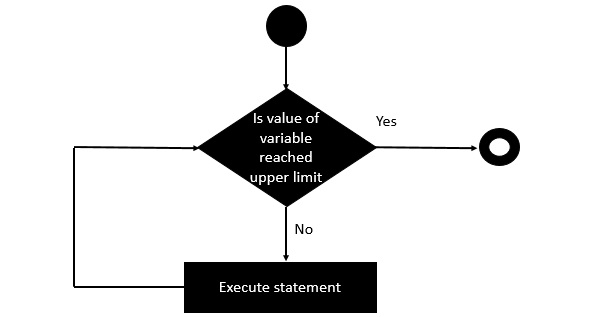# Clojure - Dotimes Statement

The ‘dotimes’ statement is used to execute a statement ‘x’ number of times.

## Syntax

Following is the general syntax of the doseq statement.

```(dotimes (variable value)
statement)
```

Where value has to be a number which indicates the number of times the loop needs to be iterated.

Following is the diagrammatic representation of this loop.## Example

Following is an example of the ‘doseq’ statement.

```(ns clojure.examples.hello
(:gen-class))

;; This program displays Hello World
(defn Example []
(dotimes [n 5]
(println n)))
(Example)
```

In the above example, we are using the dotimes statement to repeat the number if times the println statement is executed. And for each iteration it also increments the value the variable n.

## Output

The above code produces the following output.

```0
1
2
3
4
```
clojure_loops.htm